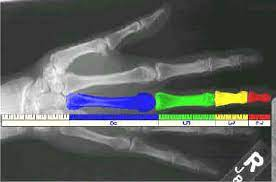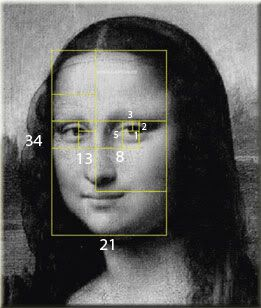Guide into Sacred Geometry

Overview:

The Golden Ratio + the curvature of space

The Golden Ratio + You

Your place in the great cosmic dance: Nassim scaling law

Sacred Geometry 101: Fundamental geometric structures in spacetime and space tiling

Seed of life

Vector equilibrium

Platonic solids

__________The Golden Ratio, Phi + the curvature of space________

Most of us have heard the word Fibonacci or heard about the golden ratio. But what do we really know about these principles?

Or how about why these patterns stretch across scales of dimensions from subatomic to the cosmic!

What many also do not know is that these principles can be found in all growing systems (and remember the universe is expanding) and all biological systems.

Before we go further let’s look at what this ratio is and how we find it.

The Fibonacci Sequence is the series of numbers:

0, 1, 1, 2, 3, 5, 8, 13, 21, 34, ...

The next number is found by adding up the two numbers before it:

• the 2 is found by adding the two numbers before it (1+1),
• the 3 is found by adding the two numbers before it (1+2),
• the 5 is (2+3),
• and so on!

Example: the next number in the sequence above is 21+34 = 55

Here is a longer list:              0, 1, 1, 2, 3, 5, 8, 13, 21, 34, 55, 89,144,233,377,610,987, 1597, 2584, 4181,

Makes A Spiral

When we make squares with those values as widths, and we draw a curved line arching from the opposite corner to the other,

we see a very familiar shape: The Spiral.   This fundamental shape is found everywhere in nature - across all scales of dimensions!Why does this shape appear in our reality in different scales of dimensions?  To answer that question, first lets look at The Golden Ratio and how it relates to the Fibonacci numbers.

When we take any two successive (one after the other) Fibonacci Numbers, their ratio is very close to the Golden Ratio "φ" which is approximately 1.618034...

In fact, the larger the pair of Fibonacci Numbers, the closer the approximation gets. Let us try a few:

 A B B / A 2 3 1.5 3 5 1.666666666... 5 8 1.6 8 13 1.625 ... ... ... 144 233 1.618055556... 233 377 1.618025751... ... ... ...

PHI, The Golden Ratio  φ

The golden ratio (symbol is the Greek letter "phi" shown at left)
is a special number approximately equal to 1.618

We find the golden ratio when we divide a line into two parts so that:

the whole length divided by the long part

is also equal to

the long part divided by the short partThis is also known as: the golden sectiongolden meangolden numberdivine proportiondivine section and golden proportion.

So we have Learned that:

The Fibonacci Sequence is a simple set of numbers that are closely associated with the Phi ratio the higher the numbers go up the sequence.

This Golden Ratio is meaningful because we find it across all scales of dimensions.

******

Lets take a closer look at this repeating pattern and look inward to see how we are, ourselves directly connected to this Principle of Sacred Geometry.

Here we see the same numerical numbers as shown in length measurements of the the digits in the skeleton of the hand:Here we have the Length of the hand to the forearm:Here we have proportions of the human face:The Phi Ratio is in your DNAThe DNA molecule, the program for all life, is based on the golden section. It measures 34 angstroms long by 21 angstroms wide for each full cycle of its double helix spiral. 34 and 21, of course, are numbers in the Fibonacci series and their ratio, 1.6190476 closely approximates phi, 1.6180339.

B-DNA has spirals in phi proportions. DNA in the cell appears as a double-stranded helix referred to as B-DNA.This form of DNA has a two groove in its spirals, with a ratio of phi in the proportion of the major groove to the minor groove, or roughly 21 angstroms to 13 angstroms.

Okay so its within us and outside of us.  So why is this phenomenon happening?

So we know this ratio is part of how our body is constructed.  Where else do we find this ratio?

In all growing systems

Snail shells, Pinecones, Sunflower, Whirlpools, Hurricanes, Spiral galaxies

Literally everywhere

WHY - WHY - WHY does this ratio exist?

The Universe is always seeking for the most efficient systems.  Think of Stars.  So incredibly efficient!  Some stars will burn for billions and billons of years!  Our universe, from the micro to the macro is constantly working towards more and more densely compacted matter, and also higher and higher systems of efficiency.

The Phi Ratio is the most efficient construction technique for our

OKAY, So what do these symbols have to do with me?

All energy events in our universe are polarized in structure.  Directional currents of energy maintain counter rotating magnetic fields due to a curvature of spacetime.  These energies form vectors in orbits.  These vectorial orbits move in coherent wave patterns forming specific geometries.  Therefor All observable phenomena in our universe is geometric!  Atomic nuclei bonding structure, electron wave clouds, Virus structures, Crystalline arrays, hexagonal vortex structure on Saturns North Pole, Pyramidal structural arrangement of galactic super clusters.

Even though these energy events have an extreme variation in size and scale, they all adhere to a geometric structure of reality.

Every physical energetic event ( an atom, a cell, you, a star) is geometrically structured through polarized rotating magnetic fields.  These energies oscillate in geometric vectorial orbits that reduce unnecessary travel time, increase energy efficiency and maximize space tiling (use the maximum amount of the local space available).

Let’s look at some of these fundamental geometric structures and discuss what the are.  One thing that I want you to visualize as you look at these “objects” and “structures” is that these are in fact 3d energy vectorial pathways.  These 2d designs represent something physically real, they represent very real physical objects that exist in our universe.

Space Tiling: The job of the universe is to pack as much information (energy in formations) in as little of space as possible.  This fact precedes all further geometries, as the role of geometry is to find the underlying space tiling limitations of energy in spacetime.

We begin with the Seed of Life